﻿ Higgs gamma-gamma WW channel decay process

# Higgs bosons really exist in the accelerators ?

## What is Higgs particle ?

You may have seen some Higgs particle's "concrete" images on TV or something.
Basically, they try to explain Higgs to you using "real" and "concrete" things, which are not true.
For example, Higgs is like the sea, in which electrons and quarks are "floating".
Or Higgs is "resistance", in which particles "feel" their masses.
But in fact, the correct answer is Higgs is only "math".
( Unfortunately, they try NOT to tell you this truth. )

(Fig.1) What is Higgs ? Sea ? Resistance ? or only Math ?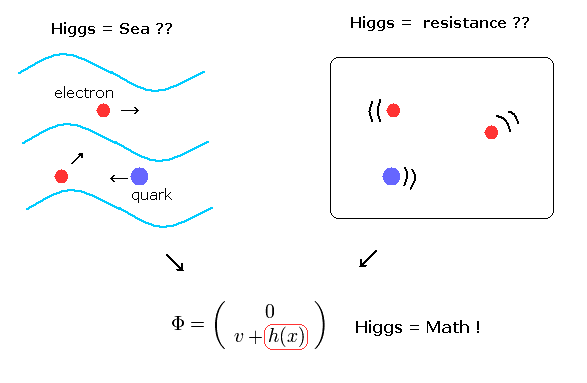Here we show the reason why Higgs is necessary for the standard model in brief.

## Why we need Higgs boson ?

Why very "abstract" Higgs boson was born ? That reason exists in the relativity, I think.
The relativistic restriction is the strongest in all theories.
According to the relativity, all things must look the same from the observer moving at arbitrary velocities.
So we had to give up "concrete" images and rely only on very "abstract" math.

In these very limited conditions, they turned their attentions to "gauge transformation" + "symmetry (= gauge invariance )".
( Unfortunately, these are just "math", and have no clear images at all. )

## The current physics gives only nonphysical particles.

(Eq.1) ↓ This is electron ? (= kinetic + mass m terms )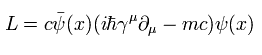## Gauge (= θ ) lacks physical reality.

(Eq.2) gauge (= θ ) transformation  ← Not physics !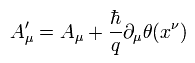## Photon (= A ) is just nonphysical math with No shape.

(Eq.3) Antisymmetric tensor Fμν of Maxwell's Lagrangian doesn't change by gauge transformation.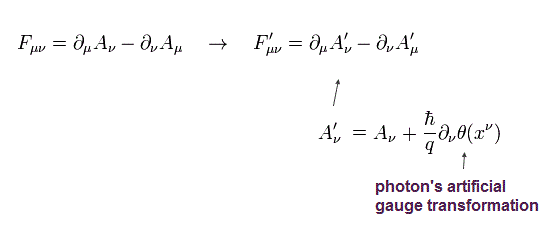(Eq.4) Maxwell's Lagrangian L doesn't change (= invariant) under gauge transformation.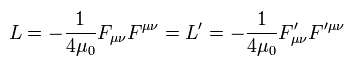## Symmetry and Higgs lack reality.

### [ Nonphysical gauge transformation → mass term is redundant ? → Higgs is needed ? ]

(Fig.2) Photon's "mass term" is NOT invariant under gauge transformation.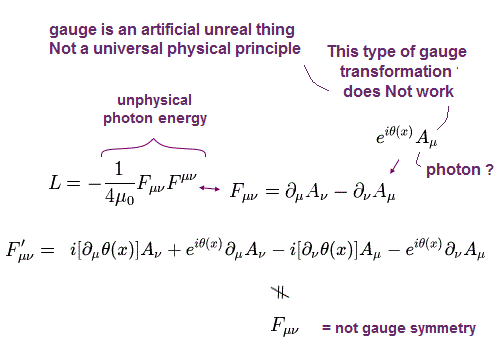As shown in Fig.2, the usual Maxwell's Lagrangian of Eq.4 is invariant when we substitute Eq.2 into Eq.3 and Eq.4.
Because the gauge term of θ is cancelled out.
Basically the gauge θ is a unrealistic thing, so the gauge should NOT appear openly.
But if the Maxwell's Lagrangian includes additional photon's mass term like Fig.2, this term changes when we substitute Eq.2 into this mass term.
( So in this case, gauge appears openly. = mass term is Not invariant under gauge transformation. )

Photons and gluons have no mass, so we don't need to add this annoying mass term to Maxwell's Lagrangian.
But very heavy W and Z bosons have this serious problem. ( Of course, only mathematical reason. )
To solve this problem, we suppose the masses of W and Z bosons are zero, and introduce a new concept of Higgs field to give them mass keeping "symmetry".

As shown in this page, left-handed and right-handed electrons or quarks are completely different things ( one is "matrix", and another is "number" ).
This strange definition comes from the asymmetrical experimental results of beta decay.
Furthermore, up and down quarks, electrons and neutrinos are mixed in the electroweak unification theory.
So we can not decide "definite" mass terms of them.
As a result, electrons and quarks must have no mass, either, and we rely on Higgs mechanism to give the masses to them.
( As you notice, the most important things are "mass terms" + gauge invariance (= symmetry ). This is only a rule. )

"Higgs wind" ? or Higgs is moving with the earth ?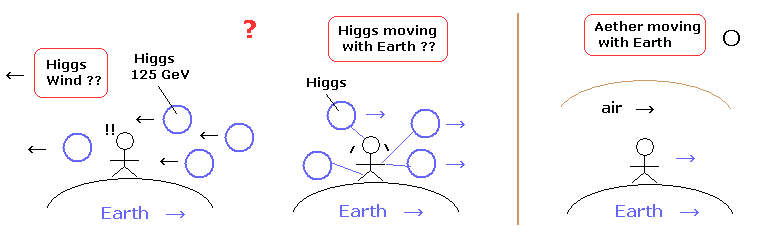As I explain later, Higgs need to exist in every place where fermions such as quarks and electrons are moving around.
Because in relativistic QFT the interaction terms among Higgs and fermions include the common variable xμ of them.
So, for example, at the same place as down quark inside the nucleus, heavy Higgs particle (= about 125 times proton ) always exist !
Why the quark inside nucleus and moving electrons can NOT be obstructed by these very heavy Higgs particles ?
This is very unreasonable.
In the "symmetry" theory, if Higgs don't exist at the same place as fermions, all fermions lose mass.
Because the fermions must NOT have any mass to keep symmetry.
( This is a rule, so if you believe in standard model, you need to obey it. )

You may think Higgs are unstable and vanish, so they don't obstruct the fermion's movement.
But in fact, there are too many Higgs particles in the vacuum ( of course, at the same places as fermions ).
Because Higgs scalar fields include the following terms of Higgs (= h ).

## Higgs (= h ) has No physical figure.

Terms of Higgs particles (= h )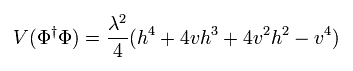## Weak boson (= W ) lacks physical reality.

To understand Higgs production in the accelerators, we first need to understand how W and Z bosons interact with other particles.
Maxwell's Lagrangian which gives ordinary Maxwell's equations are

(Eq.5) Maxwell's Lagrangian. (= "number" )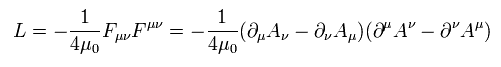As shown in this page, Lagrangian of W bosons are more complicated than Maxwell's one.
Of course, they are similar to each other, except one is "number", another is "matrix".
The fact that we must rely on "matrix" means these theories are only "mathematical" (= not real ) things.

(Eq.6) Weak boson Lagrangian. (= "2 × 2 matrix" )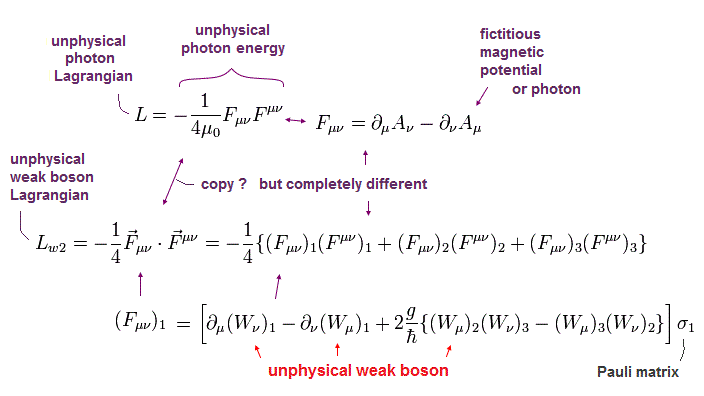Antisymmetric tensor Fμν of weak bosons are
(Eq.7)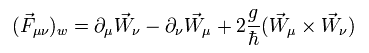For example, "1" component of Eq.7 is
(Eq.8)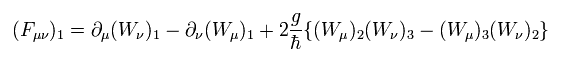Substituting every component of Eq.7 (Eq.8) into Lagrangian of Eq.6, we get various interaction terms in weak and Z bosons.
We change 1-3 components of W bosons and B field into W± bosons, Z boson, and photon (= A ) in the unification theory.

(Eq.9) Transformation into W± bosons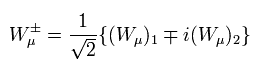(Eq.10) Unification and transformation into Z and A bosons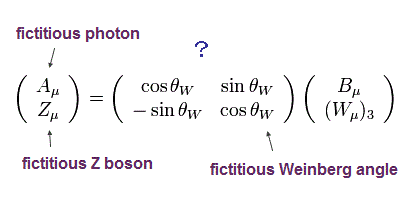In this theory, we need to substitute W±, Z and A bosons into B and three kinds of W bosons of the following Lagrangian,
(Eq.11)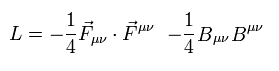A part of the terms we can get from Eq.11 are

(Eq.12) W bosons and A (= photon ) interaction term.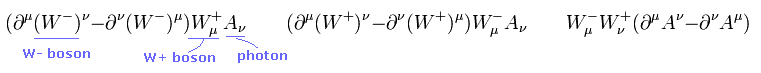(Eq.13) W and Z bosons interaction term.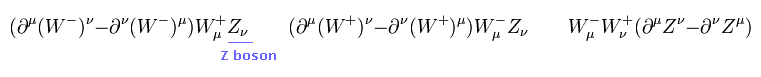Using Feynman diagram, the interaction terms of Eq.12 and Eq.13 can be expressed as

(Fig.3) Feynman diagram of W, Z and A boson interaction terms.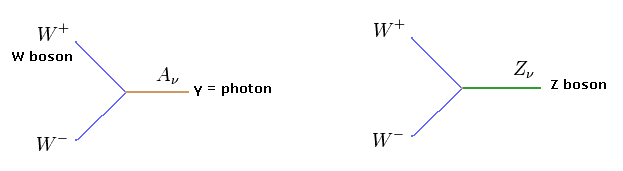In relativistic QFT and QED, interaction terms and Feynman diagrams are all we can use when considering interactions between particles.
So we can use only very abstract and limited "mathematical" tools like these in QFT.
( This is a main reason why we lose "reality" in the present relativistic QFT and particle physics. )
Using the interaction of Eq.12, the accelerator can create a pair of W bosons like electron + positron → photon (= A ) → WW bosons.
Of course, W bosons can NOT be observed directly, so this interpretation completely relies on the standard model.

Other terms of Eq.11 are

(Eq.14) W bosons interaction term.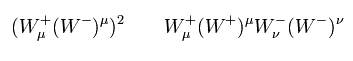(Eq.15) WW and ZZ bosons interaction term.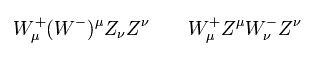(Eq.16) WW and ZA bosons interaction term.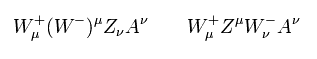(Eq.17) WW and AA bosons interaction term.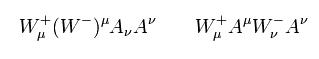Combinig these various interaction terms, we can think about various interaction processes.
So even if just one of these processes shows positive results, it needs to be reconsidered, I think.
( Because it is possible that the "intermediate" particles which can not be observed directly may be just an "illusion". )

## Higgs (= h ) gives mass to W boson ?  ← Not physics

As shown in this page ( Eq.86- Eq.102 ), if we use the Higgs field (= h(x) ) of
(Eq.18)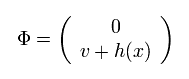where "v" is a constant in the vacuum, and h(x) is a Higgs boson.
This parameter "v" can NOT be gotten from the standard model itself.

We can get the terms of
(Eq.19)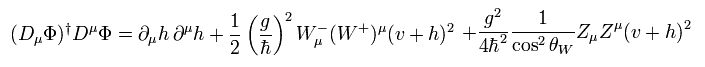In Eq.19, the mass terms of W and Z bosons are ( × 1/2 )
(Eq.20)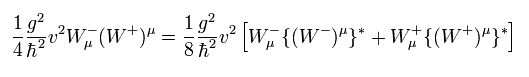and
(Eq.21)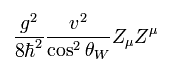From the definition of Eq.10 and considering the upper component of Eq.18 is zero, the photon (= A ) mass term doesn't appear in Higgs field of Eq.19.
This is important, but a little "artificial" manipulation, I think.

Other terms of Eq.19 include

(Eq.22) W, Z bosons and Higgs interaction term.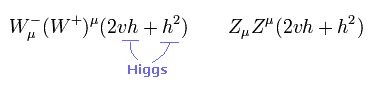Some terms of E.22 can be expressed as

(Fig.4) Feynman diagram of W, Z and Higgs boson interaction terms.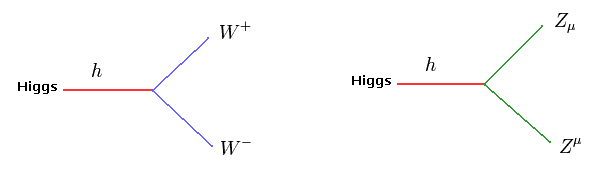Fig.4 interactions show the WW and ZZ channels decay processes in the accelerators.
Of course, we can NOT observe these intermediate particles such as W, Z, and Higgs directly.
These existences completely depend on the standard model.
( So if the standard model changes, this interpretation changes. )
For example, we just analyze the motion of final products such as electrons, electromagnetic waves and neutrinos.
( Not seeing W, Z, and Higgs. )

## The current physics gives only nonphysical Higgs.

According to the standard model, if Higgs doesn't exist, all fermions such as electrons and quarks have no mass, which means all things vanish !
Like the weak bosons, we need to introduce new interaction Lagrangians of fermions and Higgs.
For example, the Lagrangian which generates quark's mass is

(Eq.23) Lagrangian of quarks and Higgs.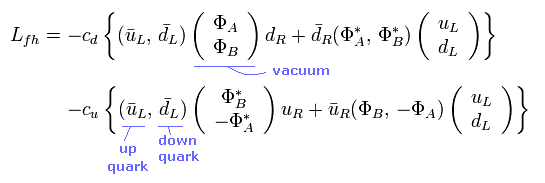where the vacuum is
(Eq.24)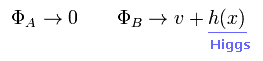uL and dL of Eq.23 mean "left-handed up quark" and "left-handed down quark", respectively.
The important point is that Eq.23 and Eq.24 are only "definitions" (= rules ), which are NOT "natural" things.
Substituting Eq.24 into Eq.23, we get
(Eq.25)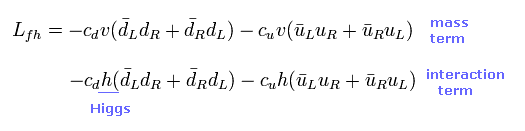where
(Eq.26)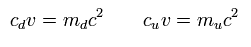md and mu mean the masses of down and up quarks.

The first line of Eq.25 mean the mass terms of down and up quarks.
And the second line of Eq.25 mean the interaction terms among Higgs (= h ) and quarks.
So the strength of these interactions are related to their masses.
But when we define Eq.23, it is natural that we get these results.

"Infinite" and "very heavy" Higgs particles really exist ? or only "illusions" ?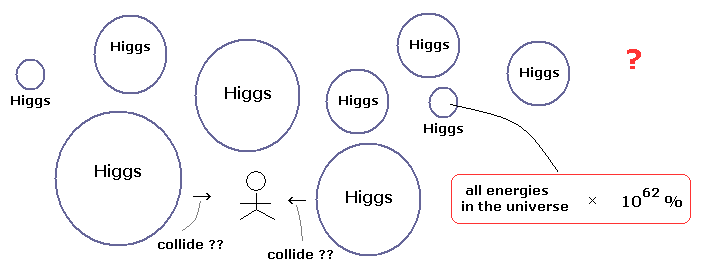If we try to think that the interactions between fermions and Higgs cause their masses (= resistance ), Higgs need to exist in every place where fermions are moving around.
How these heavy Higgs particles (= about 125 times proton ) can feel fermion's motion, not obstructing their moving directions ?
This is very strange.

The standar model can NOT predict the masses of quarks.
So we need to decide the value "v" of the vacuum and the constants cd, cu ONLY from the experiments.
This is the weak point of the standard model, which can NOT predict them at all.

Using Feynman diagram, Eq.25 can be expressed as

(Fig.5) Feynman diagram of "quarks" and "Higgs boson" interaction.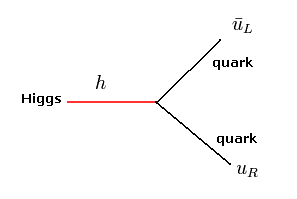Fig.5 is also an important process in the accelerator.
But we can not observe these quarks and Higgs directly.
( This interpretation depends on the standard model. )

## Quark, gluon is nonphysical math with No shape.

From this page ( Eq.103- Eq.109 ), we get the interaction term of gluon (= G ) and fermions (= quarks, ψ )

(Eq.27) Interaction terms of gluons and quarks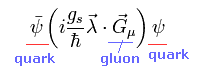Feynman diagram of Eq.27 is

(Fig.5) Feynman diagram of "quarks" (= q ) and "gluon" (= G ) interaction.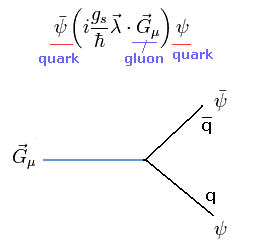Gluons have no mass, so they don't need to use Higgs mechanism to keep symmetric.
This means the interaction term between gluon and Higgs doesn't exist.
The process of Fig.5 is important in the accelerators which uses proton-proton collisions.
Because according to the standard model, almost all energies (= mass ) of the proton come from "gluon" binding energies.
Of course, if the standard model is wrong, this interpretation can not be used.

According to Einstein's mass energy relations, every mass energy is equal to the energy of mc2.
Why the gluon energy (= proton mass ) has NO relation with Higgs ??
The idea of "mass" is NOT a common thing ??
Do you feel some "mathematical" and "artificial" tricks in this difference between proton mass (= gluon ) and fermion's mass ?

## Higgs decay in accelerator lacks physical reality.

Combinig these interaction terms, we can think of a lot of Higgs production and decay processes.
So, to put it accurately, all these patterns need to be confirmed in the experiments.
But it seems that they think only one of them is enough for the confirmation of Higgs existence. This is strange.
And furthermore, the standard model includes many parameters, which can NOT be gotten from the standard model itself.

From this page ( Eq.47 and Eq.72 ), we can consider the interactions among fermions, photons (= A ), and W bosons.

(Fig.6) Feynman diagram of interactions among fermions, W boson and photons.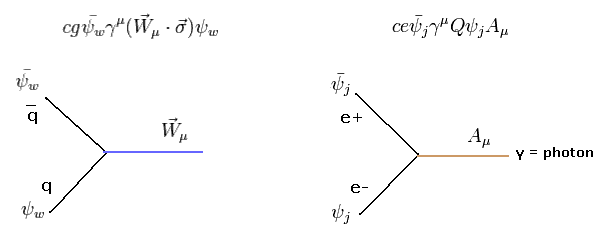The interactions among charged particles and photons originally exist in Dirac Lagrangian.
And from the beta decay, weak bosons interact with fermions such as quarks, electrons, and neutrinos.

Fig.6 is important in the accelerator which uses electron (e-) and positron (e+) collision.

(Fig.7) gamma-gamma channel decay process of Higgs.Fig.7 is one example of the decay process in which proton-proton collision → Higgs → two photons (= gamma γ rays ).
Of course, as shown in the upper section, there are many kinds of patterns in the intermediate process.
So Fig.7 is only one of them.

(Fig.8) Proton- antiproton collision to generate Higgs.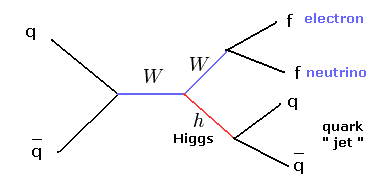Fig.8 shows one example in which particle and antiparticle collision generates Higgs.
But the quarks, Higgs, and W bosons can NOT be taken out and observed directly.
So, all we can do is to observe the final products and believe this process is correct.
( Quarks can not be isolated, so they are only observed as "jets" with other particles. )

### [ Higgs existence really can be confirmed by these experiments ? ]

You may often hear the news such as " the probability of finding Higgs is about 99 % or something".
The important point is how we decide the background state without Higgs.
Because we need to compare Higgs with "estimated" no Higgs state to decide Higgs existence.
All we can do is to estimate these "no Higgs" states from background patterns of various energy states.
Of course, if we make mistakes in deciding these no Higgs state, the interpretation of the experimental results change.

It is a very difficult task to distinguish between them, because it is said that trillion collisions generates only a few potential Higgs particles.

After the news which report the finding of "God" particle Higgs, you probably want to know more about Higgs particles and question about their nature somewhere.
When I read various blogs and bulletin boards on the internet, the answers to these questions are extremely "vague", so I am afraid that the present science can NOT get out of these vague and abstract "math" states forever.

For example, we want to know about
" All fermions and W, Z bosons have no mass, and their mass is caused by the interaction with the vacuum (= Higgs field ) around them. "
" Why photons (= electromagnetic wave) or gluons can NOT feel their (= Higgs field ) existence at all ? "
" Gravity is caused by the exchange of gravitons. So gravitons do NOT feel the fermions (or W, Z bosons) directly, and instead gravitons can feel Higgs field around them ?
This is very difficult to imagine. How do they distinguish them correctly ? "

(Fig.9) What is the "true" relationship between gravity and mass ?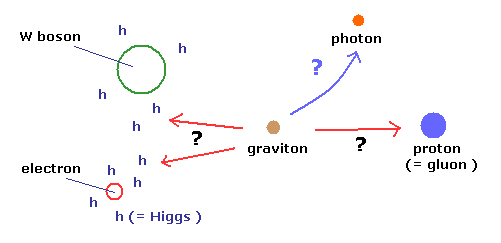As shown in Fig.9, the "clever" graviton can distinguish electrons, W bosons, protons and photons correctly ?
Because in case of electrons and W bosons, the gravitons act on the Higgs fields around them ( NOT on electrons themselves directly ).
But in case of protons, the gravitons act on the protons themselves.
Furthermore, according to the general relativity, the photon (= light) moving direction is bent by the gravity.
But the photon has NO mass. How can these very "clever" gravitons feel their differences ??
As you notice, this theory (= standard model ) is a very unnatural one.

(Fig.10) What is "Vacuum" ? → Nothing ? or "Infinite" particles ?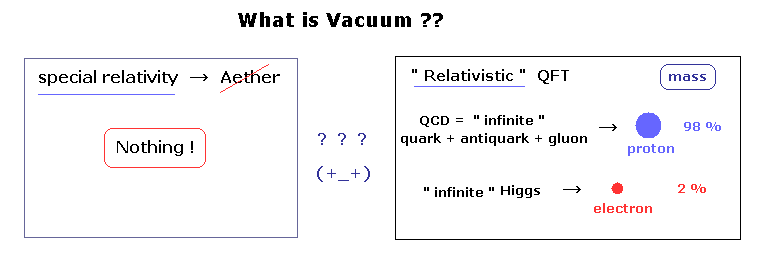As you know, according to the special relativity, the "aether" is denied, which means the vacuum contains "nothing".
Strange to say, in the relativistic quantum field theory (QFT), which combines special relativity and quantum theory, the vacuum MUST contain infinite and many kinds of particles ! The situation becomes much worse.
So the realtivistic QFT completely ignores the special relativity, though it relies on the relativity.

You may hear Higgs finding news. But according to the QFT, the mass involved in Higgs accounts for only 2 % of all things.
98 % of all masses are caused by quantum chromodynamics (= QCD ).
According to QCD and "many-world like" path integral theory, infinite pairs of quarks and antiquarks are always generated and vanish via gluons.
These aggregations via gluons are said to cause 98% mass such as protons.

But for example, the electrons has NO relations with gluon, so Higgs is also indispensable for the standard model and QCD.
Do you think these theories sound too good to be true ?
They denied "aether" wind, but these infinite particles in the QFT vacuum cause "wind" like aether ?
It is more natural that we admit "aether" moving with earth than these infinite "strange" and "unrealistic" particles.

(Eq.28) Vacuum in Higgs fields(Eq.29) Mass of fermions.As shown in Eq.28 and Eq,29, fermions mass is caused by the vacuum "v".
This means the Higgs mechanism uses a kind of "aether", which causes their mass.
But they try NOT to admit this fact. How do you think about it ?
So, this vacuum value "v" causes "wind" like "aether wind" ?

Of course, this mechanism is linked to Higgs scalar equations which contains Higgs momentum and mass terms.
If Higgs and Higgs interaction terms do not exist in every place, we can NOT generate Higgs particles in the accelerators.
And when new W bosons and a pair of electron and positron are generated in the experiments, Higgs particles need to exist at the same places to give their mass.
So these very heavy Higgs can obstruct the fermion's movement ?
And "infinite" QCD particles also obstruct their movement ?
Do you think these theories are more unnatural than aether theory ?

If you question about these things on some blogs or bulletin boads on the internet, they probably can not answer them clearly.
Because as I said many times, these theories such as standard model and QFT are "unrealistic" and only "mathematical" things (= "Shut up and calculate !" ).

Einstein did NOT admit these strange quantum theory throughout his life, as seen in the debate with Bohr.
But on the other hand, Einstein admitted Bohr model and de Broglie theory.
He said "Bohr model must be correct", when he heard Bohr model is valid also in the helium ion (He+).2012/7/4 updated. Feel free to link to this site.